Atmos. Meas. Tech., 13, 1921–1935, 2020
https://doi.org/10.5194/amt-13-1921-2020
Atmos. Meas. Tech., 13, 1921–1935, 2020
https://doi.org/10.5194/amt-13-1921-2020

Research article 16 Apr 2020

Research article | 16 Apr 2020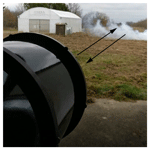# A new lidar inversion method using a surface reference target applied to the backscattering coefficient and lidar ratio retrievals of a fog-oil plume at short range

A new lidar inversion method using a surface reference target applied to the backscattering coefficient and lidar ratio retrievals of a fog-oil plume at short range
Florian Gaudfrin1,2,3, Olivier Pujol2, Romain Ceolato1, Guillaume Huss3, and Nicolas Riviere1 Florian Gaudfrin et al.
• 1ONERA, DOTA, Université de Toulouse, 31055 Toulouse, France
• 2Univ. Lille, UMR 8518 - LOA - Laboratoire d'Optique Atmosphérique, 59000 Lille, France
• 3LEUKOS SARL, 37 Rue Henri Giffard, 87280 Limoges, France

Correspondence: Florian Gaudfrin (florian.gaudfrin@onera.fr) and Nicolas Riviere (nicolas.riviere@onera.fr)

Abstract

In this paper, a new elastic lidar inversion equation is presented. It is based on the backscattering signal from a surface reference target (SRT) rather than that from a volumetric layer of reference (Rayleigh molecular scatterer) as is usually done. The method presented can be used when the optical properties of such a layer are not available, e.g., in the case of airborne elastic lidar measurements or when the lidar–target line is horizontal Also, a new algorithm is described to retrieve the lidar ratio and the backscattering coefficient of an aerosol plume without any a priori assumptions about the plume. In addition, our algorithm allows a determination of the instrumental constant. This algorithm is theoretically tested, viz. by means of simulated lidar profiles and then using real measurements. Good agreement with available data in the literature has been found.

Share
1 Introduction

Atmospheric aerosols are liquid or solid particles dispersed in the air of natural (volcano, biomass burnings, desert, ocean) or anthropogenic origins. They play an important role not only in cloud formation , in radiative forcing and more generally for research on the climate change but also in the context of air quality and public health . Their size varies from the nanometer to the millimeter scale (Robert, unpublished). However, a large majority of aerosols have a size between 0.01 and 3 µm for which scattering is dominant in the optical domain. The Mie theory is often used, at least statistically (i.e., for a large population of random sized aerosols), although aerosols are not always spherical. The optical backscattering and extinction properties of aerosols are mainly related to their shape , size distribution , concentration and chemical composition, which is based to their nature (dust, maritime or urban). Lidars are active remote sensing instruments suitable for aerosol detection and characterization over kilometric distances during both day and nighttime.

The optical properties of aerosols are obtained by means of inversion methods using the simple scattering lidar equation. In the 1980s, a stable one-component formulation adapted to lidar applications was proposed by . It has then been extended to a two-component formulation, viz. separating molecular and aerosol contributions, by and . The elastic lidar equation is an ill-posed problem in the sense of since one searches for extinction and backscattering coefficients with only a single observable. Several assumptions are therefore required in order to invert the lidar equation.

• i.

A calibration constant is usually determined from a volumetric layer of the upper atmosphere as a reference target . This calibration layer can be very high in altitude; it has recently been moved from the around 32 km to around 36–39 km for the CALIPSO spaceborne lidar in order to reduce uncertainties in the inversion procedure . This volume is considered to be made only of pure molecular constituents whose optical scattering properties are well known (Rayleigh regime). The molecular backscattering coefficient is generally estimated from the standard model of the atmosphere . However, poor estimates of the reference or low signal-to-noise ratios (SNRs) can lead to severe uncertainties in the retrieved extinction and backscattering coefficients. Few sensitivity studies have been performed to evaluate such uncertainties . Spatial averaging around the volume of reference in addition to time averaging is thus recommended to increase SNR.

• ii.

Lidar ratio is constant over the distance range of measurements . This is also an important source of errors in the retrieval values. Some studies have proposed a variable lidar ratio under the form of a power-law relationship between the extinction and backscattering coefficients, but such a method requires a priori knowledge of the medium under study (Klett1985).

• iii.

The molecular contribution along the lidar line is known. It is estimated, as for the backscattering coefficient, by means of temperature and pressure vertical profiles, using either the standard model of the atmosphere or radio soundings (Jäger2005).

In the case of elastic lidar inversion, the most critical parameter is the lidar ratio (LR). It depends on the wavelength (in vacuum) and the microphysics, morphology and size of the particles . The LR ranges from 20 to 100 sr at 532 nm according to the aerosol origins (maritime, urban, dust particles and biomass burning). It is therefore difficult to assume an a priori value for LR inasmuch as this information is to be found rather than given.

Several alternatives have been analyzed to constrain the inversion procedure while relaxing assumption (ii). These alternatives are based on the determination of the optical thickness, the one which consists of coupling lidar and sun photometer measurements being the most widely used. The measured optical thickness is then used to constrain extinction profiles . A second alternative consists of combining elastic lidar and Raman measurements in order to get the optical depth as a function of range . In a third technique, the optical depth is retrieved from elastic lidar measurements with different zenith angles . It is worth indicating that coupling lidar and sun photometer measurements is possible only for daytime, while Raman measurements are carried out preferentially at nighttime in order to increase the SNR. A fourth method consists of the determination of the optical thickness and lidar ratio of transparent layers located above opaque clouds that are used as reference for calibration in the inversion procedure . This method is used for down-looking lidar measurements capable of measuring depolarization ratios. However, the method is limited to lidar systems in non-polarized detection and for lidar measurements for which clouds cannot be used as a reference. A fifth approach consists of the determination of the optical thickness of the atmosphere from the sea surface echo by combining lidar and radar measurements . This method has been used to find the lidar ratio and the optical depth of aerosol layers over oceans .

Another limitation of ground-based lidar measurements is related to the overlap function, which strongly impacts (and prevents) observation close to the instrument, i.e., in the lowest layers of the troposphere where aerosols are emitted. Different studies have proposed to modify the overlap function analytically or empirically . Some lidar devices are also equipped with a second telescope of higher overlap at short range . However, current lidar systems are not adapted enough to the monitoring and characterization of volumetric targets at short range, for instance in the industrial context or more generally, for anthropogenic activities .

To meet new industrial emission control requirements and the very recently emitted anthropogenic aerosols characterization, we have developed a short-range lidar of high spatial resolution . The lidar inversion cannot be performed by means of the classical Klett–Fernald equation, because the reference layer used for the inversion is either impossible to access (horizontal lidar measurements and sky-to-ground lidar airborne measurements) or inaccessible because of a finite lidar range. In the present paper, a modification of the conventional lidar equation is proposed in order to perform lidar inversions using a surface reference target (SRT) at relatively short range (rmax≈100 m). Precisely, a unified lidar equation for surface and volumetric scattering media is suggested, and it is then used for a new inversion equation, inspired by the Klett–Fernald equation, using a SRT.

Also a new technique to retrieve the lidar ratio without using any sun photometer, Raman or radar measurements is presented and applied to an aerosol plume. This new inversion technique is both assessed theoretically and experimentally using real lidar measurements. A discussion and a conclusion follow and close the present paper.

2 Unified lidar equation for surface and volumetric scattering media

Currently, lidar inversion methods use a volumetric layer of the upper atmosphere (higher than an altitude of 8 km above ground level) as a reference target. This volume is considered as being free of aerosols and made only of pure molecular constituents whose optical scattering properties are known. In our approach, we propose using a SRT of known bidirectional reflectance distribution function (BRDF) fr,λ (sr−1) .

This requires modification of the usual lidar equation in order to make it suitable for both surface and volumetric targets.

For the single-scattering lidar equation, for which light has undergone only one scattering event, the measured backscattered power, at range r, can be written in a general way, viz. by considering both a surface target and a volumetric target , as

$\begin{array}{}\text{(1)}& \begin{array}{rl}{\mathcal{P}}_{\mathit{\lambda }}\left(r,\phantom{\rule{0.125em}{0ex}}{\mathit{\theta }}_{i}\right)& ={\mathcal{P}}_{\mathrm{p},\mathit{\lambda }}\phantom{\rule{0.125em}{0ex}}\frac{c{\mathit{\tau }}_{\mathit{\lambda }}}{\mathrm{2}}\phantom{\rule{0.125em}{0ex}}\frac{{A}_{\mathrm{ef}}}{{r}^{\mathrm{2}}}\phantom{\rule{0.125em}{0ex}}\left\{{\mathit{\beta }}_{\mathit{\lambda }}\left(r\right)+\frac{\mathrm{2}}{c{\mathit{\tau }}_{\mathit{\lambda }}}\phantom{\rule{0.125em}{0ex}}{f}_{\mathrm{r},\mathit{\lambda }}\right\\\ & \left({r}_{\mathrm{s}},\phantom{\rule{0.125em}{0ex}}{\mathit{\theta }}_{i}\right){F}_{\mathrm{cor}}}\phantom{\rule{0.125em}{0ex}}{T}_{\mathit{\lambda }}^{\mathrm{2}}\left(r\right)\phantom{\rule{0.125em}{0ex}}{\mathit{\xi }}_{\mathit{\lambda }}\left(r\right)\phantom{\rule{0.125em}{0ex}}{\mathit{\eta }}_{\mathit{\lambda }},\end{array}\end{array}$

where 𝒫p,λ (W) is the peak power of the laser source, $c\approx \mathrm{3}×{\mathrm{10}}^{\mathrm{8}}\phantom{\rule{0.125em}{0ex}}\mathrm{m}\phantom{\rule{0.125em}{0ex}}{\mathrm{s}}^{-\mathrm{1}}$ is the Einstein constant, τλ (s) is the laser pulse duration (full width at half maximum) and Aef (m2) is the telescope effective receiving area θi (rad) the angle between the normal eigenvector to the SRT and the incident beam direction. It should be noted that in the particular case of a Lambertian surface, ${f}_{\mathrm{r},\mathit{\lambda }}\left({r}_{\mathrm{s}},\phantom{\rule{0.125em}{0ex}}{\mathit{\theta }}_{i}\right)$ can be easily expressed by spectral bidirectional reflectance factor ρλ from ρλ cos θiπ . However, the general form of BRDF (fr,λ) will be considered later in this work in order to not restrict the approach to specific cases. Also, the SRT is located at range rs, with ξλ the dimensionless overlap function and ηλ the dimensionless optical efficiency of the whole receiver. 𝒫p,λ is a rectangular-shaped pulse in the volumic lidar equation (Measures1992), viz. the ratio between the pulse energy and τλ. In the case of lidar measurements on a SRT, the backscattered peak power is not proportional to 𝒫p,λ. A corrective factor Fcor depending on the real shape of the laser pulse is thus introduced. In the present case, ${\mathcal{P}}_{\mathrm{p},\mathit{\lambda }}^{\mathrm{G}}={\mathcal{P}}_{\mathrm{p},\mathit{\lambda }}^{\mathrm{s}}\phantom{\rule{0.125em}{0ex}}{F}_{\mathrm{cor}}$, with ${\mathcal{P}}_{\mathrm{p},\mathit{\lambda }}^{\mathrm{G}}$ and ${\mathcal{P}}_{\mathrm{p},\mathit{\lambda }}^{\mathrm{s}}$ the peak powers of a Gaussian-shaped and a square laser pulse, respectively. Conservation of the pulse energy between these two kinds of pulses gives ${F}_{\mathrm{cor}}=\mathrm{2}\phantom{\rule{0.125em}{0ex}}\left(\mathrm{ln}\mathrm{2}/\mathit{\pi }{\right)}^{\mathrm{1}/\mathrm{2}}$ . The factor does not apply to the volume part of the lidar equation because, in this last part, the pulse profile is assumed to be constant over a rate duration τλ. This approximation cannot be made for the backscatter peak of a SRT, because the backscattered energy is not integrated over a volume.

In Eq. (1), ${T}_{\mathit{\lambda }}^{\mathrm{2}}$ the back and forth atmospheric transmission throughout the environment between the lidar source and range r is

$\begin{array}{}\text{(2)}& {T}_{\mathit{\lambda }}\left(r\right)=\mathrm{exp}\left[-\underset{\mathrm{0}}{\overset{r}{\int }}{\mathit{\alpha }}_{\mathit{\lambda }}\left(x\right)\phantom{\rule{0.125em}{0ex}}\mathrm{d}x\right],\end{array}$

with αλ (m−1) being the total extinction coefficient at wavelength λ and range r, as ${\mathit{\alpha }}_{\mathit{\lambda }}={\mathit{\alpha }}_{\mathrm{b},\mathit{\lambda }}+{\mathit{\alpha }}_{\mathrm{a},\mathit{\lambda }}$. The subscripts “b” and “a” refer, respectively, to the contribution of the background (molecules and aerosols) and to the contribution of the aerosol volumetric target under investigation. The total backscattering coefficient β (m−1 sr−1) is ${\mathit{\beta }}_{\mathit{\lambda }}={\mathit{\beta }}_{\mathrm{b},\mathit{\lambda }}+{\mathit{\beta }}_{\mathrm{a},\mathit{\lambda }}$, with the same meaning as just above for the subscripts. By definition, the corresponding lidar ratios are LR${}_{\mathrm{b},\mathit{\lambda }}\left(r\right)={\mathit{\alpha }}_{\mathrm{b},\mathit{\lambda }}/{\mathit{\beta }}_{\mathrm{b},\mathit{\lambda }}$ and LR${}_{\mathrm{a},\mathit{\lambda }}\left(r\right)={\mathit{\alpha }}_{\mathrm{a},\mathit{\lambda }}/{\mathit{\beta }}_{\mathrm{a},\mathit{\lambda }}$.

The fundamental quantity measured by the lidar instrument is a voltage V (in volts) which is proportional to the backscattered power as follows: ${V}_{\mathit{\lambda }}\left(r\right)={R}_{\mathrm{v},\mathit{\lambda }}\phantom{\rule{0.125em}{0ex}}{\mathcal{P}}_{\mathit{\lambda }}\left(r\right)$, where Rv,λ is the detection constant (V W−1) which determines the light–voltage conversion. It can be written using the instrumental constant as follows: ${C}_{\mathrm{ins}}={R}_{\mathrm{v},\mathit{\lambda }}{K}_{s}$ (V m3), where ${K}_{s}={\mathcal{P}}_{\mathrm{p},\mathit{\lambda }}c{\mathit{\tau }}_{\mathit{\lambda }}{A}_{\mathrm{ef}}\mathit{\eta }/\mathrm{2}$. In the literature, Cins is obtained from 𝒫λ, while, herein, it comes from the voltage and therefore takes into account all the emission, collection, detection and acquisition chain.

In the sequel, for better readability, the subscript λ and θi will not be written thereafter.

The range-corrected lidar signal Vλ(r)r2 is as follows:

$\begin{array}{}\text{(3)}& \begin{array}{rl}S\left(r\right)& ={C}_{\mathrm{ins}}\phantom{\rule{0.125em}{0ex}}\left({\mathit{\beta }}_{\mathrm{a}}\left(r\right)+{\mathit{\beta }}_{\mathrm{b}}\left(r\right)+{f}_{\mathrm{r}}\frac{\mathrm{2}}{c\mathit{\tau }}\phantom{\rule{0.125em}{0ex}}{F}_{\mathrm{cor}}\right)\\ & \mathrm{exp}\left\{-\mathrm{2}\underset{\mathrm{0}}{\overset{r}{\int }}\left[{\mathit{\alpha }}_{\mathrm{a}}\left(x\right)+{\mathit{\alpha }}_{\mathrm{b}}\left(x\right)\right]\phantom{\rule{0.125em}{0ex}}\mathrm{d}x\right\}.\end{array}\end{array}$

To remove the α dependence in the exponential term, we will replace αa and αb with LRa and LRb, respectively, and introduce the term

$\begin{array}{}\text{(4)}& {\mathrm{LR}}_{\mathrm{a}}\left(r\right)\phantom{\rule{0.125em}{0ex}}\mathrm{exp}\left\{-\mathrm{2}\underset{\mathrm{0}}{\overset{r}{\int }}{\mathit{\beta }}_{\mathrm{b}}\left(x\right)\left[{\mathrm{LR}}_{\mathrm{a}}\left(x\right)-{\mathrm{LR}}_{\mathrm{b}}\left(x\right)\right]\phantom{\rule{0.125em}{0ex}}\mathrm{d}x\right\},\end{array}$

as detailed in Ansmann and Müller (2004). With such modifications, the final lidar equation for surface and volumetric scatterers can thus be written as

$\begin{array}{}\text{(5)}& \begin{array}{rl}S& \left(r\right)\phantom{\rule{0.125em}{0ex}}{\mathrm{LR}}_{\mathrm{a}}\left(r\right)\phantom{\rule{0.125em}{0ex}}\mathrm{exp}\left\{-\mathrm{2}\underset{\mathrm{0}}{\overset{r}{\int }}{\mathit{\beta }}_{\mathrm{b}}\left(x\right)\left[{\mathrm{LR}}_{\mathrm{a}}\left(x\right)-{\mathrm{LR}}_{\mathrm{b}}\left(x\right)\right]\phantom{\rule{0.125em}{0ex}}\mathrm{d}x\right\}\\ & ={C}_{\mathrm{ins}}\left[Y\left(r\right)+{\mathrm{LR}}_{\mathrm{a}}\left(r\right)\frac{\mathrm{2}{f}_{\mathrm{r}}}{c\mathit{\tau }}\phantom{\rule{0.125em}{0ex}}{F}_{\mathrm{cor}}\right]\phantom{\rule{0.125em}{0ex}}\mathrm{exp}\left[-\mathrm{2}\underset{\mathrm{0}}{\overset{r}{\int }}Y\left(x\right)\phantom{\rule{0.125em}{0ex}}\mathrm{d}x\right],\end{array}\end{array}$

with $Y\left(r\right)={\mathrm{LR}}_{\mathrm{a}}\left(r\right)\phantom{\rule{0.125em}{0ex}}\left[{\mathit{\beta }}_{\mathrm{b}}\left(r\right)+{\mathit{\beta }}_{\mathrm{a}}\left(r\right)\right]$.

Thereafter, in order to highlight the expression to solve, it is convenient to define background-corrected transmission factor as

$\begin{array}{}\text{(6)}& D\left(\mathrm{0},r\right)=\mathrm{exp}\left\{-\mathrm{2}\underset{\mathrm{0}}{\overset{r}{\int }}{\mathit{\beta }}_{\mathrm{b}}\left(x\right)\phantom{\rule{0.125em}{0ex}}\left[{\mathrm{LR}}_{\mathrm{a}}\left(x\right)-{\mathrm{LR}}_{\mathrm{b}}\left(x\right)\right]\phantom{\rule{0.125em}{0ex}}\mathrm{d}x\right\},\end{array}$

and W(r)=S(r)LRa(r)D(r). Finally, Eq. (3) becomes

$\begin{array}{}\text{(7)}& \begin{array}{rl}W\left(r\right)& ={C}_{\mathrm{ins}}\phantom{\rule{0.125em}{0ex}}\left[Y\left(r\right)+{\mathrm{LR}}_{\mathrm{a}}\left(r\right)\frac{\mathrm{2}{f}_{\mathrm{r}}}{c\mathit{\tau }}\phantom{\rule{0.125em}{0ex}}{F}_{\mathrm{cor}}\right]\\ & \mathrm{exp}\left[-\mathrm{2}\underset{\mathrm{0}}{\overset{r}{\int }}Y\left(x\right)\phantom{\rule{0.125em}{0ex}}\mathrm{d}x\right].\end{array}\end{array}$

We will now introduce the lidar framework adapted to the radiative parameter retrieval of a volumetric scattering medium with a known SRT.

3 New lidar inversion technique

The current Klett–Fernald inversion method consists of determining Cins using the high atmosphere as a reference and fixing the LRa a priori. In this paper, Cins is determined by means of a SRT located at range rs. So,

$\begin{array}{}\text{(8)}& {C}_{\mathrm{ins}}=\frac{c\mathit{\tau }}{\mathrm{2}{f}_{\mathrm{r}}\phantom{\rule{0.125em}{0ex}}{F}_{\mathrm{cor}}}\phantom{\rule{0.125em}{0ex}}W\left({r}_{\mathrm{s}}\right)\phantom{\rule{0.125em}{0ex}}\frac{\mathrm{1}}{{\mathrm{LR}}_{\mathrm{a}}\left({r}_{\mathrm{s}}\right)}\phantom{\rule{0.125em}{0ex}}\mathrm{exp}\left[\mathrm{2}\underset{\mathrm{0}}{\overset{{r}_{\mathrm{s}}}{\int }}Y\left(x\right)\phantom{\rule{0.125em}{0ex}}\mathrm{d}x\right].\end{array}$

It is worth mentioning that LRa(rs) is the lidar ratio just before the SRT and Y(rs)=0 (only at the SRT). Also, obviously, for r<rs, fr=0. Inserting Eq. (8) into Eq. (7) gives

$\begin{array}{}\text{(9)}& W\left(r\right)=\frac{c\mathit{\tau }}{\mathrm{2}{f}_{\mathrm{r}}\phantom{\rule{0.125em}{0ex}}{F}_{\mathrm{cor}}}\phantom{\rule{0.125em}{0ex}}\frac{W\left({r}_{\mathrm{s}}\right)}{{\mathrm{LR}}_{\mathrm{a}}\left({r}_{\mathrm{s}}\right)}\phantom{\rule{0.125em}{0ex}}Y\left(r\right)\phantom{\rule{0.125em}{0ex}}\mathrm{exp}\left[\mathrm{2}\underset{r}{\overset{{r}_{\mathrm{s}}}{\int }}\phantom{\rule{0.125em}{0ex}}Y\left(x\right)\phantom{\rule{0.125em}{0ex}}\mathrm{d}x\right].\end{array}$

This equation applies only before the SRT and can be solved by integrating both sides from r to rs (). The exponential term is (see Appendix) as follows:

$\begin{array}{}\text{(10)}& \mathrm{exp}\left[\mathrm{2}\underset{r}{\overset{{r}_{\mathrm{s}}}{\int }}Y\left(x\right)\phantom{\rule{0.125em}{0ex}}\mathrm{d}x\right]=\mathrm{1}+\frac{\mathrm{4}{f}_{\mathrm{r}}\phantom{\rule{0.125em}{0ex}}{F}_{\mathrm{cor}}\phantom{\rule{0.125em}{0ex}}{\mathrm{LR}}_{\mathrm{a}}\left({r}_{\mathrm{s}}\right)}{c\mathit{\tau }\phantom{\rule{0.125em}{0ex}}W\left({r}_{\mathrm{s}}\right)}\phantom{\rule{0.125em}{0ex}}\underset{r}{\overset{{r}_{\mathrm{s}}}{\int }}W\left(x\right)\phantom{\rule{0.125em}{0ex}}\mathrm{d}x.\end{array}$

Plugging Eq. (10) into Eq. (9), we obtain in the following:

$\begin{array}{}\text{(11)}& Y\left(r\right)=W\left(r\right)\phantom{\rule{0.125em}{0ex}}{\left[\frac{c\mathit{\tau }\phantom{\rule{0.125em}{0ex}}W\left({r}_{\mathrm{s}}\right)}{\mathrm{2}{f}_{\mathrm{r}}\phantom{\rule{0.125em}{0ex}}{F}_{\mathrm{cor}}\phantom{\rule{0.125em}{0ex}}{\mathrm{LR}}_{\mathrm{a}}\left({r}_{\mathrm{s}}\right)}+\mathrm{2}\underset{r}{\overset{{r}_{\mathrm{s}}}{\int }}W\left(x\right)\phantom{\rule{0.125em}{0ex}}\mathrm{d}x\right]}^{-\mathrm{1}}.\end{array}$

Using the definitions of Y(r) and W(r) (see above), βa(r) can be written as

$\begin{array}{}\text{(12)}& \begin{array}{rl}{\mathit{\beta }}_{\mathrm{a}}\left(r\right)& =S\left(r\right)D\left(\mathrm{0},r\right)\phantom{\rule{0.125em}{0ex}}\left[\frac{c\mathit{\tau }\phantom{\rule{0.125em}{0ex}}S\left({r}_{\mathrm{s}}\right)D\left(\mathrm{0},{r}_{\mathrm{s}}\right)}{\mathrm{2}{f}_{\mathrm{r}}\phantom{\rule{0.125em}{0ex}}{F}_{\mathrm{cor}}}+\mathrm{2}\underset{r}{\overset{{r}_{\mathrm{s}}}{\int }}S\left(x\right)\\ & {\mathrm{LR}}_{\mathrm{a}}\left(x\right)D\left(\mathrm{0},x\right)\phantom{\rule{0.125em}{0ex}}\mathrm{d}x{\right]}^{-\mathrm{1}}-{\mathit{\beta }}_{\mathrm{b}}\left(r\right).\end{array}\end{array}$

This equation can be written as

$\begin{array}{}\text{(13)}& \begin{array}{rl}{\mathit{\beta }}_{\mathrm{a}}\left(r\right)& =S\left(r\right)D\left({r}_{\mathrm{s}},r\right)\phantom{\rule{0.125em}{0ex}}\left[\frac{c\mathit{\tau }\phantom{\rule{0.125em}{0ex}}S\left({r}_{\mathrm{s}}\right)}{\mathrm{2}{f}_{\mathrm{r}}\phantom{\rule{0.125em}{0ex}}{F}_{\mathrm{cor}}}+\mathrm{2}\underset{r}{\overset{{r}_{\mathrm{s}}}{\int }}S\left(x\right){\mathrm{LR}}_{\mathrm{a}}\left(x\right)\\ & D\left({r}_{\mathrm{s}},x\right)\phantom{\rule{0.125em}{0ex}}\mathrm{d}x{\right]}^{-\mathrm{1}}-{\mathit{\beta }}_{\mathrm{b}}\left(r\right).\end{array}\end{array}$

Then, by definition of the lidar ratio, we deduct αa(r)=LRa(r)βa(r). Equation (13) is similar to the one defined by , except that βb in Eq. (13) also contains the contribution of the aerosol background.

Assuming that the properties of the SRT are well known, the most critical parameter is LRa(r). Giving a value for LRa requires a priori knowledge of the volumetric target under study, whereas the main objective of lidar remote sensing is precisely to characterize the medium investigated. A priori information is always a topic of discussions, and it causes more or less severe flaws in lidar measurements.

Equation (13) can also be applied to the important context of airborne observations. In this case, it is necessary to know the ground BRDF.

## 3.2 Determination of LRa and βa: methodology

The objective is to first retrieve βa(r) and LRa – and then to deduce αa(r) – without any a priori knowledge about the medium considered. Two lidar measurements are performed as follows: the first one (signal Vs) in the absence of the volumetric aerosol medium of interest and a subsequent one (signal Vsv) in its presence. The SRT is obviously present for both measurements. The two measurements should be performed close in time in order to avoid the background environment evolving too much. The experimental setup of these lidar measurements is illustrated in Fig. 1.

By definition, the half-logarithmic ratio of Ss and Ssv corresponds to the total extinction of the volumetric media under study: ${\mathit{\alpha }}_{\mathrm{tot}}=\mathrm{ln}\left[{S}_{s}\left({r}_{\mathrm{s}}\right)/{S}_{\mathrm{sv}}\left({r}_{\mathrm{s}}\right)\right]/\mathrm{2}$. Using Ss, Cins can be determined independently of the volumetric medium of interest as follows:

$\begin{array}{}\text{(14)}& {C}_{\mathrm{ins}}=\frac{c\mathit{\tau }}{\mathrm{2}{f}_{\mathrm{r}}\phantom{\rule{0.125em}{0ex}}{F}_{\mathrm{cor}}}\phantom{\rule{0.125em}{0ex}}{S}_{s}\left({r}_{\mathrm{s}}\right)\phantom{\rule{0.125em}{0ex}}\mathrm{exp}\left[\mathrm{2}\underset{\mathrm{0}}{\overset{{r}_{\mathrm{s}}}{\int }}{\mathit{\alpha }}_{\mathrm{b}}\left(x\right)\phantom{\rule{0.125em}{0ex}}\mathrm{d}x\right],\end{array}$

which is Eq. (8) with αa=0. Cins and αtot are used in objective functions to retrieve LRa and are assumed to be uniform – r independent. The first objective function is

$\begin{array}{}\text{(15)}& {\mathit{\epsilon }}_{\mathrm{1}}=\left|\underset{{r}_{\mathrm{0}}}{\overset{{r}_{\mathrm{s}}}{\int }}{\mathit{\alpha }}_{\mathrm{a}}\left(x\right)\phantom{\rule{0.125em}{0ex}}\mathrm{d}x-{\mathit{\alpha }}_{\mathrm{tot}}\right|,\end{array}$

where αa is the retrieved profile of extinction using Eq. (13) and LRa. The medium is assumed to be at a range of full overlap (r>r0), so that αtot must correspond to the integrated extinction. A second objective function,

$\begin{array}{}\text{(16)}& {\mathit{\epsilon }}_{\mathrm{2}}=\left|\underset{{r}_{\mathrm{0}}}{\overset{{r}_{\mathrm{s}}}{\int }}\left[{S}_{\mathrm{sv}}\left(x\right)-{S}_{\mathrm{sim}}\left(x\right)\right]\phantom{\rule{0.125em}{0ex}}\mathrm{d}x\right|,\end{array}$

is introduced in order to minimize the difference between Ssv and the simulated signal Ssim obtained from the retrieved βa and αa and from Cins.

The methodology is presented in Fig. 2. The molecular background contribution is computed from pressure and temperature data as in , while the aerosol background contribution is estimated by means of radiative transfer codes, e.g., MATISSE or MODTRAN . Another solution consists of using a realistic value of the visibility 𝒱 (km−1) and Koschmieder's relation at 550 nm (maximum human eye sensitivity): 𝒱αb≈3.9.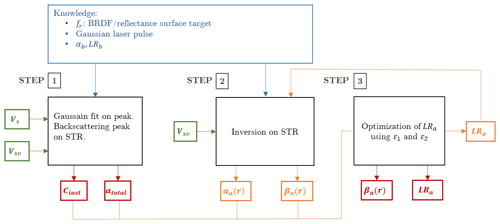Figure 2Diagram illustrating how the inversion algorithm allows one to retrieve the βa(r) and LRa without assumptions about the volume medium of interest. In green are the lidar signals inputs; in orange are the intermediate calculations during the optimization procedure, and in red are the code outputs.

The signals Vs and Vsv are introduced in the inversion procedure, which is organized around three main steps (Fig. 2):

1. A Gaussian fit is first applied to the backscattered signal from the SRT, i.e., Vs(rs) and Vsv(rs), which gives the amplitude of the backscattering, the position of this peak and its width in position. From these Gaussian models, one can obtain αtot (from its definition; see above) and Cins from Eq. (14). Note: when the target is tilted with respect to the lidar-target line, the backscatter peak of surface target will not be symmetrical. Another fit should be used as a lognormal function.

2. A first lidar inversion is realized using Eq. (13) with LRa=50 sr at the beginning of the inversion procedure. This value has been chosen because it corresponds to the average LRa data from the literature. For that, the Gaussian model Vsv obtained at step 1 is used for signal S(rs) in Eq. (13). A first range-profile βa(r) is thus obtained at the end of this second step.

3. The above βa(r) and LRa allow us to determine αa(r) whose r integration is then compared with αtot in the minimization procedure of Eq. (15). At each iteration, the LRa is modified in order to reduce ε1. The new βa(r), LRa and αa(r) values are then used to compute a simulated lidar signal Ssim whose comparison with Ssv is minimized according to Eq. (16). In this algorithm, the iterative procedure ends when ${\mathit{\epsilon }}_{\mathrm{1}}+{\mathit{\epsilon }}_{\mathrm{2}}\le {\mathrm{10}}^{-\mathrm{6}}$ is reached. The use of 19 iterations is generally enough, depending on the first value of LRa introduced initially (step 2). At the end of this step, one thus obtains final βa(r), αa(r) and LRa. The minimization procedure used is the one implemented by . Equation (15) is the most important since it determines the rapidity of convergence. Equation (16) is helpful but not critical.

4 Theoretical behavior of the retrieval procedure

## 4.1 Theoretical lidar signals

The inversion method described above is tested using theoretical lidar signals generated by PERFALIS (PERFormence Assesment for LIdar Systems; ). As summarized in Table 1, the simulated atmosphere is composed of three layers and a SRT of BRDF ${f}_{\mathrm{r}}=\mathrm{0.20}/\mathit{\pi }$ located at rs=100 m. Pressure and temperature are uniform (1040 hPa and 290 K), and the continental aerosol background is chosen so that it corresponds to 𝒱=47 km . In addition, ${\mathit{\beta }}_{\mathrm{b}}=\mathrm{9.97}×{\mathrm{10}}^{-\mathrm{6}}\phantom{\rule{0.125em}{0ex}}{\mathrm{m}}^{-\mathrm{1}}\phantom{\rule{0.125em}{0ex}}{\mathrm{sr}}^{-\mathrm{1}}$ and LRb=118.56 sr. The signal Vs is generated from the background components and the SRT, while the signal Vsv is generated considering an aerosol plume aerosol between 20 and 30 m (second layer). The plume backscatter coefficient is ${\mathit{\beta }}_{\mathrm{a}}=\mathrm{7.14}×{\mathrm{10}}^{-\mathrm{5}}\phantom{\rule{0.125em}{0ex}}{\mathrm{m}}^{-\mathrm{1}}\phantom{\rule{0.125em}{0ex}}{\mathrm{sr}}^{-\mathrm{1}}$ and LRa=70 sr. Multiple scattering is assumed to be negligible. For dense atmosphere and wider field of view, Eq. (1) has to be corrected by an appropriate factor in order to consider higher orders of scattering events.

Table 1Input optical parameters of the scene used in the lidar simulator (PERFALIS code) as illustrated in Fig. 1.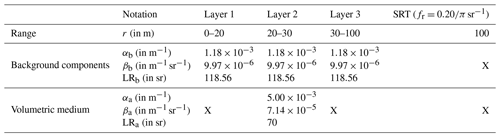Inversion methods are generally applied to averaged signals in order to increase the SNR. In lidar remote sensing, the noise can be, approximately, considered as a white Gaussian noise . In order to assess the impact of noise in the inversion method (see Sect. 3), a Gaussian noise of null mean value and a standard deviation of $\mathrm{1.5}×{\mathrm{10}}^{-\mathrm{5}}\phantom{\rule{0.125em}{0ex}}\mathrm{a}.\mathrm{u}.$ is introduced in the theoretical lidar signals. Figure 3 displays the theoretical noised signals Vs and Vsv. As expected, because of light extinction by the plume, Vsv(rs) is lower than Vs(rs) by 9 %. Four datasets are then generated, with respectively, an averaging over 20, 50, 100 and 200 signals, from Vs and Vsv, and, in addition, a fifth signal without noise is considered (Fig. 4).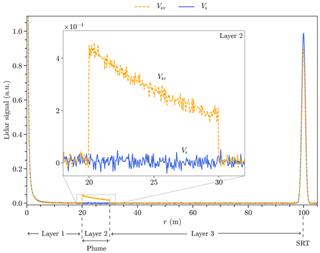Figure 3Theoretical noised lidar signals from a SRT Vs (blue line) and in the presence of an aerosol plume Vsv (orange dashed line). Simulations have been performed at 532 nm with molecular and continental aerosol background contributions.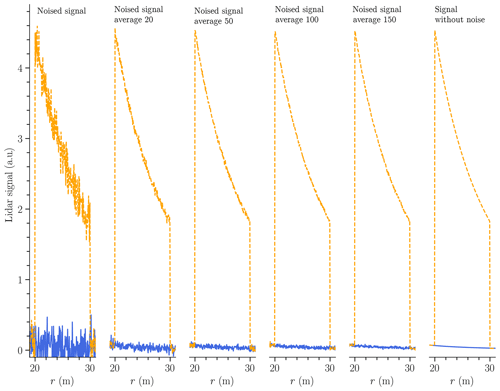Figure 4Lidar datasets used in the inversion method. In blue (orange), the lidar signal in the absence (presence) of the volumetric media under study.

## 4.2 Noise impact on βa and LRa retrievals

LRa is retrieved using Eq. (15). In addition to the six-lidar dataset described above, four different conditions of inversion are considered. In condition 1 the exact data of the background components are used as an input in the inversion algorithm. For conditions 2 and 3, βb is over- and underestimated by 20 % compared to the data used to generate the theoretical signals. In conditions 1 to 3, the inversion technique is performed over the entire signal range. Condition 4 is the same as condition 1, but the aerosol plume is spatially delimited. Table 2 summarizes the four conditions for the six datasets. It is worth mentioning that noised lidar signals obviously result in noised retrieved βa(r). Thus, to quantify the performance of the inversion technique, we consider the average value $\stackrel{\mathrm{‾}}{{\mathit{\beta }}_{\mathrm{a}}}$ of the plume. The retrieved value of LRa can be directly compared to the theoretical value.

Figure 5 displays $\stackrel{\mathrm{‾}}{{\mathit{\beta }}_{\mathrm{a}}}$ for the six datasets and the four inversion conditions. It varies from $\mathrm{7.11}×{\mathrm{10}}^{-\mathrm{5}}$ to $\mathrm{7.22}×{\mathrm{10}}^{-\mathrm{5}}\phantom{\rule{0.125em}{0ex}}{\mathrm{m}}^{-\mathrm{1}}\phantom{\rule{0.125em}{0ex}}{\mathrm{sr}}^{-\mathrm{1}}$, which means an error of approximately 1 % in comparison to the theoretical value. Conditions 2 and 3 result in a translation of the corresponding curve of ±0.4 % with respect to the curve associated with condition 1 because of the over- and underestimation of 20 % introduced in βb. The performance is better for condition 4 whatever the dataset is, since the maximum error is 0.5 % for noised signals. The spatially bounded aerosol layer is often applied in inversion methods and seems to herein improve the inversion method.

For signal lidar without noise, ${\stackrel{\mathrm{‾}}{\mathit{\beta }}}_{\mathrm{a}}$ is not exactly equal to the theoretical value, maybe because of numerical computation errors in the inversion algorithm. Such a numerical error is about 0.12 % (condition 1) and 0.04 % (condition 4).

Figure 6 is similar to Fig. 5 but considers LRa. One obtains values ranging from 66 to 74 sr, with a maximum error of 5 % compared to the theoretical value. In conditions 1, 2 and 3, using averaged noised signals has no consequence on the retrieved value of LRa, contrary to what was obtained for ${\stackrel{\mathrm{‾}}{\mathit{\beta }}}_{\mathrm{a}}$.

In condition 1, the maximum error is 2.1 %. The graphs corresponding to conditions 2 and 3 are translated with respect to the graph under to condition 1 by about ±3 % and permuted by the same but for ${\stackrel{\mathrm{‾}}{\mathit{\beta }}}_{\mathrm{a}}$. Nevertheless, the errors remain low with a maximum of 5 % (condition 2) if 50 signals are averaged. However, under condition 4, the LRa is much better for averaged signals and remains quite good for the noised signal (not averaged) with an error rate of 0.6 %. Again, it seems that the spatial limitation of the plume increases the accuracy of the retrieval LRa. Condition 1 remains, however, efficient for noised signals since deviation is below 2.1 %. In the case of the lidar signal without noise, the retrieved LRa values are not exactly equal to the theoretical LRa values; numerical computation errors are about 0.13 % (condition 1) and 0.05 % (condition 4). An error of ±20 % in βb introduced initially will result in an under- or overestimation LRa by ±3 %. Condition 4 is preferable for retrieving LRa.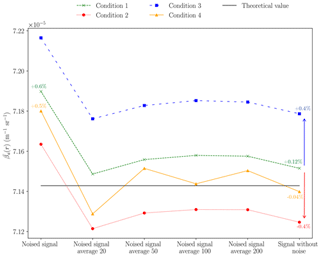Figure 5Retrieved ${\stackrel{\mathrm{‾}}{\mathit{\beta }}}_{\mathrm{a}}$ for six datasets and four different conditions of inversion.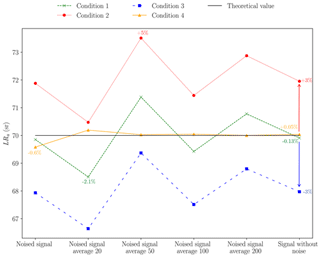Figure 6Retrieved LRa for six datasets and four different conditions of inversion.

Note that the formalism and methodology adopted here to retrieve the lidar ratio are efficient as long as the peak backscattering of the SRT is present on the lidar signal. The method has been evaluated, in this paper, for short range, around 100 m, because our research focus on application at this range. However, the algorithm developed does not present any limit with respect to the range provided that measurements are made below 1 km of range (this value depends of the power of laser sources) with respect to our applications. However, at first sight there is no limit to the application of the method for measurements at longer ranges such as those at more than 1 km.

## 4.3 Plume optical property retrieval

The above study allowed us to test the new inversion method on noised signals, for different conditions of inversion, as a function of the number of signals averaged. Thereafter, lidar inversion was performed by considering a spatially bounded plume and 100 signals for averaging. This last condition has been chosen because it corresponds to the number of signals available in less than 0.1 s with our lidar system (see Sect. 5). The theoretical results obtained by the inversion method with 100 averaged signals is also quite good (see above). Figure 7 displays the retrieved βa if a theoretical lidar signal is introduced as a first guess. Table 3 lists the retrieved ${\stackrel{\mathrm{‾}}{\mathit{\beta }}}_{\mathrm{a}}$ and LRa. Compared to theoretical values, errors are less than 0.7 % for LRa and below 0.1 % for ${\stackrel{\mathrm{‾}}{\mathit{\beta }}}_{\mathrm{a}}$, although a peak of 2.2 % is observed at r=28.8 m.

Table 2Conditions placed on the optical properties of the background components for the inversion method.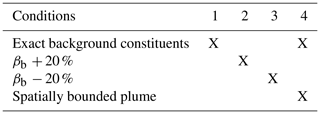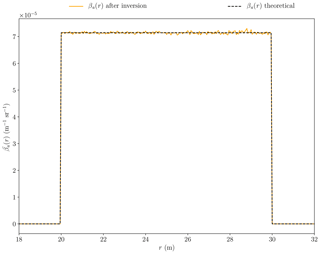Figure 7Retrieved βa(r) (orange solid line) for 100 theoretical averaged lidar signals and initial βa(r) (dark dashed line).

5 Case of real measurements

Our new inversion technique is now applied to real lidar measurements. The instrument used is named COLIBRIS (COmpact LIdar for Broadband polaRImetric multi-Static measurements; ). This lidar is able to perform short-range measurements (r0<5 m) at high spatial resolution (lower than 0.25 m). A Nd:YAG microchip laser source from HORUS, part of the LEUKOS company, is used with a pulse energy peaking at 532 nm of 7.3 µJ and a repetition rate of 1 kHz. The backscattered light is collected by a Cassegrain telescope. In the detection part, a dichroic filter for the elastic channel is used before a photomultiplier tube. The signal is digitized at a sample frequency of 3 GHz after being amplified.

## 5.1 Description of the experimental operations

The lidar measurements are performed horizontally as illustrated in Fig. 8. A Lambertian Zenithal SRT with a ${f}_{\mathrm{r}}=\mathrm{0.20}/\mathit{\pi }$ is placed 52 m away from the source. Its spectral bidirectional reflectance has been checked using laboratory bench measurements . The mean direction of the laser beam is parallel to the normal of the surface.

The repetition laser source has repetition frequency of 1 kHz. In order to increase the SNR, we preprocess the measurements from three lidar measurements:

• signal 1 – the first measurement is made by occulting the emitted laser beam to get a measure of the background scene (contribution of passive illumination);

• signal 2 – the second measurement is made by occulting the telescope to estimate the dark noise of the instrument;

• signal 3 – the last measurement is made without any occultation.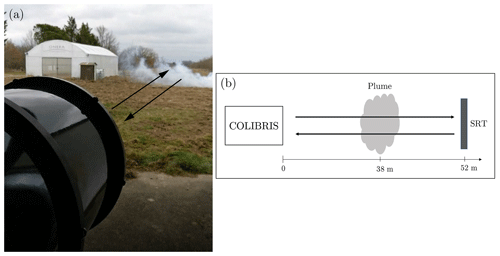Figure 8Experimental setup in a horizontal configuration. A fog-oil plume is generated between the lidar and the Lambertian SRT. (a) Photo and (b) illustration of the experimental setup with fog-oil plume.

For a given acquisition period, these three series of measured signals are averaged. The averaged signals of the background radiation and the dark noise (signals 1 and 2) are then subtracted from the signal 3 as follows: signal 3 signal 2 (signal 1 signal 2).

Figure 9 shows the lidar results on a volume/surface target over a period of 2 s. This corresponds to 2000 signals per series of measurements. During this period, we assume that the environment does not evolve significantly. The curves Vsv and Vs are the measurements in the presence and in the absence of oil smoke with SRT, respectively. The oil plume signal is visible between 37.5 and 41 m.

The high-speed sampling allows a measurement every centimeter along the line of sight. Combined with a short pulse duration of the laser source (1.7 ns), this makes it possible to highlight local variation concentrations on the order of 25 cm inside the plume with the presence of two maxima at 38 and 39 m from lidar. The peak of backscattering of the SRT is also well sampled. The signal amplitude corresponding to the backscatter of the SRT is lower for Vsv than for Vs because of the presence of the oil plume.

During measurement, the pressure, temperature and visibility are respectively 1016 hPa, 288 K and 30 km. These data are used to compute βb as described in Sect. 4.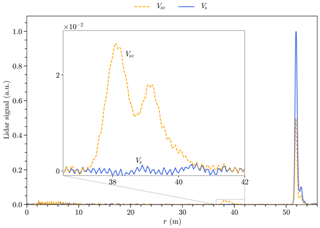Figure 9Lidar measurements of the experimental setup. In blue, (orange) the lidar signal in the absence (presence) of the oil-fog plume under study.

## 5.2 Optical property retrieval: fog-oil plume

The signals Vs and Vsv are used in the inversion procedure as described in Sects. 3 and 4. The plume is spatially bounded (condition 4).

The retrieved βa(r) is displayed in Fig. 10. In the densest range of the plume ${\mathit{\beta }}_{\mathrm{a}}\approx \mathrm{2}×{\mathrm{10}}^{-\mathrm{3}}\phantom{\rule{0.125em}{0ex}}{\mathrm{m}}^{-\mathrm{1}}\phantom{\rule{0.125em}{0ex}}{\mathrm{sr}}^{-\mathrm{1}}$. Also, the retrieved LRa is around 98 sr. According to , this value corresponds, as expected, to smoke particles (at 532 nm, the lidar ratio ranges from 80 to 100 sr).The optical properties of the oil-fog plume of experimental retrieved with inverse method are summarized in Sect. 4.

The lidar signal reproduced from the retrieved βa(r), LRa and the instrumental constant deduced from the Eq. (14) gives a standard deviation from the exact value of $\mathrm{1.5}×{\mathrm{10}}^{-\mathrm{5}}\phantom{\rule{0.125em}{0ex}}\mathrm{a}.\mathrm{u}.$ This shows the consistency and reliability of the new inversion method proposed in this paper.

Table 4Optical properties of oil-fog plume in experimental setup at 532 nm.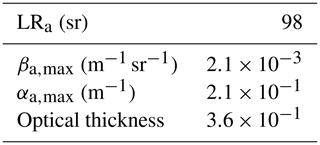6 Conclusions

In this paper, a new method has been introduced for lidar measurement inversion in a situation for which a volumetric layer (molecular Rayleigh scatterers) of the high troposphere is not available (e.g., airborne lidar observations, horizontal configuration of measurements). This method is based on a new expression of the lidar equation which allows us to use a surface reference target of a known BRDF instead of a volumetric one. This new formalism permits the inversion of short-range lidar measurements for which conventional inversion techniques cannot directly be applied. Similarly to common inversion techniques, our method requires the introduction of a background component (molecular and particulate contributions) that can either be estimated from radiative models or deduced from measurements of temperature, pressure and visibility conditions.

Also, a new algorithm has been developed to retrieve, without any a priori assumptions relative to the medium to be characterized (aerosol plume), the backscattering coefficient (βa) and lidar ratio (LRa) of an aerosol plume between the lidar and the surface target reference. In other words, our technique does not need to introduce a lidar ratio as an input for our inverse algorithm. For that, two lidar measurements are necessary, with and without the aerosol plume under consideration.

Comparing these two signals, one can retrieve the total extinction coefficient of the medium analyzed and the instrumental constant of the lidar instrument. These two pieces of information are used to constrain the inversion algorithm and finally to identify LRa.

This algorithm has been first investigated using theoretical (simulated) lidar signals. The quality of the retrieval has been assessed by introducing noise in the simulated signals and by considering various conditions of inversion differing, in particular, from one another according to the initial error introduced in the backscattering coefficient of the aerosol background. Thus, the robustness of algorithm has been shown, since in all the cases, the error on the retrieved values (viz. in βa and LRa) is less than 5 %. Also, we have found that inversion is better for spatially bounded aerosol plumes.

The inversion algorithm has then been applied to real lidar short-range measurements of an oil-fog plume. The retrieved βa and LRa of the plume agree with values found in the literature for smoke-like particles. Moreover, thanks to the determination of the instrumental constant, the measured signal has been computed from the inverted products, and an absolute error of ${\mathrm{10}}^{-\mathrm{5}}\phantom{\rule{0.125em}{0ex}}\mathrm{a}.\mathrm{u}.$ between the measure and the post-processed simulation has been encountered.

However, it is worth mentioning that the method proposed herein to find LRa has some limitations. Precisely, the sensitivity of the lidar must be sufficient to detect the signal of weakly thick or weakly backscattering plume. Indeed, since measurements are performed in the absence and in the presence of the medium, by means of a hard surface target of reference of known reflectance, the algorithm converges less easily for very weakly diffusing plumes.

The new inversion technique presented in this paper suggests new airborne lidar applications operated at low altitude from aircraft (helicopters, airplanes) but requires a priori knowledge of the reflectance of the SRT.

Even if some models exist for the BRDF of surfaces , their use seems difficult to implement because of the diversity of encountered surfaces during airborne measurements. Nevertheless, it may be possible to identify the reflectance of the ground surface by means of a spectroradiometer imager . The combination of these measurements with the herein proposed inversion method would a priori be complementary to establishing of new methods of calibration for down-looking lidar measurements (spaceborne or airborne lidars). The evaluation of the method proposed in this paper, considering the uncertainty in the target reflectance, has not been performed. It will be the topic of future works.

Appendix A

To solve Eq. (9), the exponential term can be written under another form. The method proposed by consists of integrating both members of the equation from r to rs. So,

$\underset{r}{\overset{{r}_{\mathrm{s}}}{\int }}W\left(x\right)\phantom{\rule{0.125em}{0ex}}\mathrm{d}x=\frac{c\mathit{\tau }}{\mathrm{2}{f}_{\mathrm{r}}\phantom{\rule{0.125em}{0ex}}{F}_{\mathrm{cor}}}\phantom{\rule{0.125em}{0ex}}\frac{W\left({r}_{\mathrm{s}}\right)}{{\mathrm{LR}}_{\mathrm{a}}\left({r}_{\mathrm{s}}\right)}\phantom{\rule{0.125em}{0ex}}\underset{r}{\overset{{r}_{\mathrm{s}}}{\int }}\left\{Y\left(x\right)\phantom{\rule{0.125em}{0ex}}\mathrm{exp}\left[\mathrm{2}\underset{x}{\overset{{r}_{\mathrm{s}}}{\int }}Y\left(r\right)\phantom{\rule{0.125em}{0ex}}\mathrm{d}r\right]\right\}\phantom{\rule{0.125em}{0ex}}\mathrm{d}x.$

Since

$\begin{array}{rl}\frac{\mathrm{d}}{\mathrm{d}x}& \left\{\mathrm{exp}\left[\mathrm{2}\underset{x}{\overset{{r}_{\mathrm{s}}}{\int }}Y\left(r\right)\phantom{\rule{0.125em}{0ex}}\mathrm{d}r\right]\right\}=\mathrm{2}\phantom{\rule{0.125em}{0ex}}\mathrm{exp}\left[\mathrm{2}\underset{x}{\overset{{r}_{\mathrm{s}}}{\int }}Y\left(r\right)\phantom{\rule{0.125em}{0ex}}\mathrm{d}r\right]\phantom{\rule{0.125em}{0ex}}\frac{\mathrm{d}}{\mathrm{d}x}\left[\underset{x}{\overset{{r}_{\mathrm{s}}}{\int }}Y\left(r\right)\phantom{\rule{0.125em}{0ex}}\mathrm{d}r\right]\\ & =\mathrm{2}\phantom{\rule{0.125em}{0ex}}\mathrm{exp}\left[\mathrm{2}\underset{x}{\overset{{r}_{\mathrm{s}}}{\int }}Y\left(r\right)\phantom{\rule{0.125em}{0ex}}\mathrm{d}r\right]\phantom{\rule{0.125em}{0ex}}\frac{\mathrm{d}}{\mathrm{d}x}\left[F\left({r}_{\mathrm{s}}\right)-F\left(x\right)\right]\\ & =-\mathrm{2}\phantom{\rule{0.125em}{0ex}}Y\left(x\right)\phantom{\rule{0.125em}{0ex}}\mathrm{exp}\left[\mathrm{2}\underset{x}{\overset{{r}_{\mathrm{s}}}{\int }}Y\left(r\right)\phantom{\rule{0.125em}{0ex}}\mathrm{d}r\right],\end{array}$

where F is the primitive of Y. It ensues that

$\begin{array}{rl}\underset{r}{\overset{{r}_{\mathrm{s}}}{\int }}Y\left(x\right)& \mathrm{exp}\left[\mathrm{2}\underset{x}{\overset{{r}_{\mathrm{s}}}{\int }}Y\left(r\right)\phantom{\rule{0.125em}{0ex}}\mathrm{d}r\right]\phantom{\rule{0.125em}{0ex}}\mathrm{d}x=-\frac{\mathrm{1}}{\mathrm{2}}\phantom{\rule{0.125em}{0ex}}{\left\{\mathrm{exp}\left[\mathrm{2}\underset{x}{\overset{{r}_{\mathrm{s}}}{\int }}Y\left(r\right)\phantom{\rule{0.125em}{0ex}}\mathrm{d}r\right]\right\}}_{r}^{{r}_{\mathrm{s}}}\\ & =\frac{\mathrm{1}}{\mathrm{2}}\phantom{\rule{0.125em}{0ex}}\left\{\mathrm{exp}\left[\mathrm{2}\underset{r}{\overset{{r}_{\mathrm{s}}}{\int }}Y\left(r\right)\phantom{\rule{0.125em}{0ex}}\mathrm{d}r\right]-\mathrm{1}\right\}.\end{array}$

Therefore,

$\underset{r}{\overset{{r}_{\mathrm{s}}}{\int }}W\left(x\right)\phantom{\rule{0.125em}{0ex}}\mathrm{d}x=\frac{c\mathit{\tau }}{\mathrm{4}{f}_{\mathrm{r}}\phantom{\rule{0.125em}{0ex}}{F}_{\mathrm{cor}}}\phantom{\rule{0.125em}{0ex}}\frac{W\left({r}_{\mathrm{s}}\right)}{{\mathrm{LR}}_{\mathrm{a}}\left({r}_{\mathrm{s}}\right)}\phantom{\rule{0.125em}{0ex}}\left\{\mathrm{exp}\left[\mathrm{2}\underset{r}{\overset{{r}_{\mathrm{s}}}{\int }}Y\left(r\right)\phantom{\rule{0.125em}{0ex}}\mathrm{d}r\right]-\mathrm{1}\right\}.$

Finally, the exponential term becomes

$\mathrm{exp}\left[\mathrm{2}\underset{r}{\overset{{r}_{\mathrm{s}}}{\int }}Y\left(r\right)\phantom{\rule{0.125em}{0ex}}\mathrm{d}r\right]=\mathrm{1}+\frac{\mathrm{4}{f}_{\mathrm{r}}\phantom{\rule{0.125em}{0ex}}{F}_{\mathrm{cor}}}{c\mathit{\tau }}\phantom{\rule{0.125em}{0ex}}\frac{{\mathrm{LR}}_{\mathrm{a}}\left({r}_{\mathrm{s}}\right)}{W\left({r}_{\mathrm{s}}\right)}\phantom{\rule{0.125em}{0ex}}\left[\underset{r}{\overset{{r}_{\mathrm{s}}}{\int }}W\left(r\right)\phantom{\rule{0.125em}{0ex}}\mathrm{d}r\right].$
Data availability

These data are the property of ONERA and are not accessible.

Author contributions

This work is part of the PhD thesis of FG who is the main contributor of all of the aspects (theoretical, modeling and experimental) of this article. This thesis has been supervised by NR, OP, RC and GH. OP contributed to the theoretical part of this work while experiments have been conducted by FG, RC, NR and GH. FG and RC realized the lidar system used in measurements. FG and OP wrote a main part of the article.

Competing interests

The authors declare that they have no conflict of interest.

Acknowledgements

This research work has been performed within the framework of a CIFRE grant (ANRT) for the doctoral work of Florian Gaudfrin. The lidar systems has been funded by the PROMETE project (ONERA). The laser source used in this paper for the experimental setup has been designed by Benoit Faure and Paul-Henri Pioger, who is from the LEUKOS company

Financial support

This research work has been supported within the framework of a CIFRE no 2016/0571 grant sign between LEUKOS company and French National Agency for Research and Technology (ANRT) for the doctoral work of Florian Gaudfrin. The lidar system has been funded by the PROMETE project (ONERA).

Review statement

This paper was edited by Andrew Sayer and reviewed by two anonymous referees.

References

Ackermann, J.: The extinction-to-backscatter ratio of tropospheric aerosol: A numerical study, J. Atmos. Ocean. Tech., 15, 1043–1050, https://doi.org/10.1175/1520-0426(1998)015<1043:TETBRO>2.0.CO;2, 1998. a

Anon: U.S. Standard Atmosphere, Tech. rep., NASA, Washington, DC, US, 1976. a

Ansmann, A., Riebesell, M., and Weitkamp, C.: Measurement of atmospheric aerosol extinction profiles with a Raman lidar, Opt. Lett., 15, 746–748, https://doi.org/10.1364/OL.15.000746, 1990. a

Ansmann, A. and Müller, D.: Lidar and Atmospheric Aerosol Particles, in: Range-Resolved Optical Remote Sensing of the Atmosphere, 105–141, Springer-Verlag, New York, 2004.

Ansmann, A., Wandinger, U., Riebesell, M., Weitkamp, C., and Michaelis, W.: Independent measurement of extinction and backscatter profiles in cirrus clouds by using a combined Raman elastic-backscatter lidar, Appl. Optics, 31, 7113, https://doi.org/10.1364/AO.31.007113, 1992. a

Ansmann, A., Neuber, R., Rairoux, P., and Wandinger, U.: Advances in Atmospheric Remote Sensing with Lidar, vol. 34, Springer Berlin Heidelberg, Berlin, Heidelberg, https://doi.org/10.1007/978-3-642-60612-0, 1997. a

Ansmann, A., Baldasano, J., Calpini, B., Chaikovsky, Anatoly and Flamant, P., Mitev, V., Papayannis, A., Pelon, J., Resendes, D., Schneider, J., Trickl, T., and Vaughan, G.: EARLINET: A European Aerosol Research Lidar Network, Tech. rep., 2001. a

Baltensperger, U., Dommen, J., Alfarra, M. R., Duplissy, J., Gaeggeler, K., Metzger, A., Facchini, M. C., Decesari, S., Finessi, E., Reinnig, C., Schott, M., Warnke, J., Hoffmann, T., Klatzer, B., Puxbaum, H., Geiser, M., Savi, M., Lang, D., Kalberer, M., and Geiser, T.: Combined Determination of the Chemical Composition and of Health Effects of Secondary Organic Aerosols: The POLYSOA Project, J. Aerosol Med. Pulm. D., 21, 145–154, https://doi.org/10.1089/jamp.2007.0655, 2008. a

Berk, A., Acharya, P., and Bernstein, L.: Band model method for modeling atmospheric propagation at arbitrarily fine spectral resolution, US Pat., available at: https://patents.google.com/patent/US7433806 (last access: 6 July 2019), 2008. a

Berk, A., Conforti, P., Kennett, R., Perkins, T., Hawes, F., and van den Bosch, J.: MODTRAN6: a major upgrade of the MODTRAN radiative transfer code, 9088, 90880H, International Society for Optics and Photonics, https://doi.org/10.1117/12.2050433, 2014. a

Bissonnette, L. R.: Multiple-scattering lidar equation, Appl. Optics, 35, 6449, https://doi.org/10.1364/AO.35.006449, 1996. a

Bodhaine, B. A., Wood, N. B., Dutton, E. G., and Slusser, J. R.: On Rayleigh Optical Depth Calculations, J. Atmos. Ocean. Tech., 16, 1854–1861, https://doi.org/10.1175/1520-0426(1999)016<1854:ORODC>2.0.CO;2, 1999. a

Bohlmann, S., Baars, H., Radenz, M., Engelmann, R., and Macke, A.: Ship-borne aerosol profiling with lidar over the Atlantic Ocean: from pure marine conditions to complex dust–smoke mixtures, Atmos. Chem. Phys., 18, 9661–9679, https://doi.org/10.5194/acp-18-9661-2018, 2018. a

Bréon, F.-M., Maignan, F., Leroy, M., and Grant, I.: Analysis of hot spot directional signatures measured from space, J. Geophys. Res., 107, 4282, https://doi.org/10.1029/2001JD001094, 2002. a

Bucholtz, A.: Rayleigh-scattering calculations for the terrestrial atmosphere, Appl. Optics, 34, 2765, https://doi.org/10.1364/AO.34.002765, 1995. a

Bufton, J. L.: Laser Altimetry Measurements from Aircraft and Spacecraft, P. IEEE, 77, 463–477, https://doi.org/10.1109/5.24131, 1989. a

Cattrall, C., Reagan, J., Kurt, T., and Oleg, D.: Variability of aerosol and spectral lidar and backscatter and extinction ratios of key aerosol types derived from selected Aerosol Robotic Network locations, J. Geophys. Res.-Atmos., 110, 1–13, https://doi.org/10.1029/2004JD005124, 2005. a

Ceolato, R. and Gaudfrin, F.: Short-range characterization of freshly emitted carbonaceous particles by LiDAR, in: General Meeting, Nafplio, 17–19 April 2018. a, b

Ceolato, R., Riviere, N., and Hespel, L.: Reflectances from a supercontinuum laser-based instrument: hyperspectral, polarimetric and angular measurements, Opt. Express, 20, 29413, https://doi.org/10.1364/oe.20.029413, 2012. a

Ceolato, R., Gaudfrin, F., Pujol, O., Riviere, N., Berg, M. J., and Sorensen, C. M.: Lidar cross-sections of soot fractal aggregates: Assessment of equivalent-sphere models, J. Quant. Spectrosc. Ra., 212, 39–44, https://doi.org/10.1016/j.jqsrt.2017.12.004, 2018. a

Clark, W. E. and Whitby, K. T.: Concentration and Size Distribution Measurements of Atmospheric Aerosols and a Test of the Theory of Self-Preserving Size Distributions, J. Atmos. Sci., 24, 677–687, https://doi.org/10.1175/1520-0469(1967)024<0677:CASDMO>2.0.CO;2, 1967. a

Collis, R. T. H. and Russell, P. B.: Lidar measurement of particles and gases by elastic backscattering and differential absorption, in: Laser Monitoring of the Atmosphere, 71–151, https://doi.org/10.1007/3-540-07743-X_18, 1976. a

Comeron, A., Sicard, M., Kumar, D., and Rocadenbosch, F.: Use of a field lens for improving the overlap function of a lidar system employing an optical fiber in the receiver assembly, Appl. Optics, 50, 5538, https://doi.org/10.1364/AO.50.005538, 2011. a

Dawson, K. W., Meskhidze, N., Josset, D., and Gassó, S.: Spaceborne observations of the lidar ratio of marine aerosols, Atmos. Chem. Phys., 15, 3241–3255, https://doi.org/10.5194/acp-15-3241-2015, 2015. a

DeMott, P. J., Prenni, A. J., Liu, X., Kreidenweis, S. M., Petters, M. D., Twohy, C. H., Richardson, M. S., Eidhammer, T., and Rogers, D. C.: Predicting global atmospheric ice nuclei distributions and their impacts on climate, P. Natl. Acad. Sci. USA, 107, 11217–11222, https://doi.org/10.1073/pnas.0910818107, 2010. a

Elias, T., Haeffelin, M., Drobinski, P., Gomes, L., Rangognio, J., Bergot, T., Chazette, P., Raut, J. C., and Colomb, M.: Particulate contribution to extinction of visible radiation: Pollution, haze, and fog, Atmos. Res., 92, 443–454, https://doi.org/10.1016/j.atmosres.2009.01.006, 2009. a

Fernald, F. G.: Analysis of atmospheric lidar observations: some comments, Appl. Optics, 23, 652–653, https://doi.org/10.1364/ao.23.000652, 1984. a

Fernald, F. G., Herman, B. M., and Reagan, J. A.: Determination of Aerosol Height Distributions by Lidar, J. Appl. Meteorol., 11, 482–489, https://doi.org/10.1175/1520-0450(1972)011<0482:DOAHDB>2.0.CO;2, 1972. a

Finlayson-Pitts, B. J. and Pitts, J. N. J.: The Atmospheric System, Chemistry of the Upper and Lower Atmosphere: Theory, Experiments, and Applications, 15–42, https://doi.org/10.1016/B978-012257060-5/50004-6, 2000. a

Gaudfrin, F., Ceolato, R., Pujol, O., Riviere, N., and Hu, Q.: Supercontinuum lidar for hydrometeors and aerosols optical properties determination, in: EGU General Assembly 2018, 20, 14436, 2018a. a

Gaudfrin, F., Ceolato, R., Riviere, N., Pujol, O., and Huss, G.: New technics to probe aerosols radiative properties from visible to infraredTitle, in: The 13th International IR Target and Background Modeling & Simulation Workshop, Banyuls-sur-Mer, 2018b. a, b

Gaudfrin, F., Ceolato, R., Pujol, O., and Riviere, N.: A new-lidar inversion technique using a surface reference target at short – range, in: The 29th International Laser Radar Conference, 3–6, 24–28 June, Hefei, 2019. a

Getzewich, B. J., Vaughan, M. A., Hunt, W. H., Avery, M. A., Powell, K. A., Tackett, J. L., Winker, D. M., Kar, J., Lee, K.-P., and Toth, T. D.: CALIPSO lidar calibration at 532 nm: version 4 daytime algorithm, Atmos. Meas. Tech., 11, 6309–6326, https://doi.org/10.5194/amt-11-6309-2018, 2018. a

Glickman, T. and Zenk, W.: Glossary of Meteorology, American Meteorological Society, Boston, 855 pp. 2, available at: http://oceanrep.geomar.de/6557/ (last access: 17 July 2019), 2000. a

Hadamard, J.: Théorie des équations aux dérivées partielles linéaires hyperboliques et du problème de Cauchy, Acta Math., 31, 333–380, https://doi.org/10.1007/BF02415449, 1908. a

Hall, F. F. and Ageno, H. Y.: Absolute calibration of a laser system for atmospheric probing, Appl. Optics, 9, 1820–1824, https://doi.org/10.1364/AO.9.001820, 1970. a

Halldórsson, T. and Langerholc, J.: Geometrical form factors for the lidar function, Appl. Optics, 17, 240–244, https://doi.org/10.1364/AO.17.000240, 1978. a

Haner, D. A., McGuckin, B. T., Menzies, R. T., Bruegge, C. J., and Duval, V.: Directional–hemispherical reflectance for Spectralon by integration of its bidirectional reflectance, Appl. Optics, 37, 3996, https://doi.org/10.1364/AO.37.003996, 1998. a

Hansen, J., Sato, M., and Ruedy, R.: Radiative forcing and climate response, J. Geophys. Res.-Atmos., 102, 6831–6864, https://doi.org/10.1029/96JD03436, 1997. a

Haywood, J. and Boucher, O.: Estimates of the direct and indirect radiative forcing due to tropospheric aerosols: A review, Rev. Geophys., 38, 513–543, https://doi.org/10.1029/1999RG000078, 2000. a

Hess, M., Koepke, P., Schult, I., Hess, M., Koepke, P., and Schult, I.: Optical Properties of Aerosols and Clouds: The Software Package OPAC, B. Am. Meteorol. Soc., 79, 831–844, https://doi.org/10.1175/1520-0477(1998)079<0831:OPOAAC>2.0.CO;2, 1998. a

Hoff, R. M., Bösenberg, J., and Pappalardo, G.: The GAW Aerosol Lidar Observation Network (GALION), in: International Geoscience and Remote Sensing Symposium (IGARSS-08), 27–29 March, Boston (USA), 6–11, 2008. a

Horvath, H.: On the applicability of the Koschmieder visibility formula, Atmos. Environ., 5, 177–184, https://doi.org/10.1016/0004-6981(71)90081-3, 1971. a

Hu, Y., Vaughan, M., Liu, Z., Powell, K., and Rodier, S.: Retrieving Optical Depths and Lidar Ratios for Transparent Layers Above Opaque Water Clouds From CALIPSO Lidar Measurements, IEEE Geosci. Remote S., 4, 523–526, https://doi.org/10.1109/LGRS.2007.901085, 2007. a

Hyslop, N. P.: Impaired visibility: the air pollution people see, Atmos. Environ., 43, 182–195, https://doi.org/10.1016/j.atmosenv.2008.09.067, 2009. a

Jäger, H.: Long-term record of lidar observations of the stratospheric aerosol layer at Garmisch-Partenkirchen, J. Geophys. Res.-Atmos., 110, 1–9, https://doi.org/10.1029/2004JD005506, 2005. a

Josset, D., Pelon, J., Protat, A., and Flamant, C.: New approach to determine aerosol optical depth from combined CALIPSO and CloudSat ocean surface echoes, Geophys. Res. Lett., 35, https://doi.org/10.1029/2008GL033442, 2008. a

Josset, D., Pelon, J., and Hu, Y.: Multi-Instrument Calibration Method Based on a Multiwavelength Ocean Surface Model, IEEE Geosci. Remote S., 7, 195–199, https://doi.org/10.1109/LGRS.2009.2030906, 2010a. a

Josset, D., Zhai, P.-W., Hu, Y., Pelon, J., and Lucker, P. L.: Lidar equation for ocean surface and subsurface, Opt. Express, 18, 20862, https://doi.org/10.1364/OE.18.020862, 2010b. a, b

Josset, D., Pelon, J., Garnier, A., Hu, Y., Vaughan, M., Zhai, P.-W., Kuehn, R., and Lucker, P.: Cirrus optical depth and lidar ratio retrieval from combined CALIPSO-CloudSat observations using ocean surface echo, J. Geophys. Res.-Atmos., 117, https://doi.org/10.1029/2011jd016959, 2012. a

Josset, D., Pelon, J., Pascal, N., Hu, Y., and Hou, W.: On the Use of CALIPSO Land Surface Returns to Retrieve Aerosol and Cloud Optical Depths, IEEE T. Geosci. Remote, 56, 3256–3264, https://doi.org/10.1109/TGRS.2018.2796850, 2018. a, b

Kar, J., Vaughan, M. A., Lee, K.-P., Tackett, J. L., Avery, M. A., Garnier, A., Getzewich, B. J., Hunt, W. H., Josset, D., Liu, Z., Lucker, P. L., Magill, B., Omar, A. H., Pelon, J., Rogers, R. R., Toth, T. D., Trepte, C. R., Vernier, J.-P., Winker, D. M., and Young, S. A.: CALIPSO lidar calibration at 532 nm: version 4 nighttime algorithm, Atmos. Meas. Tech., 11, 1459–1479, https://doi.org/10.5194/amt-11-1459-2018, 2018. a

Kavaya, M. J., Menzies, R. T., Haner, D. A., Oppenheim, U. P., and Flamant, P. H.: Target reflectance measurements for calibration of lidar atmospheric backscatter data, Appl. Optics, 22, 2619, https://doi.org/10.1364/AO.22.002619, 1983. a

Klett, J. D.: Stable analytical inversion solution for processing lidar returns, Appl. Optics, 20, 211–220, https://doi.org/10.1364/AO.20.000211, 1981. a, b

Klett, J. D.: Lidar inversion with variable backscatter/extinction ratios, Appl. Optics, 24, 1638, https://doi.org/10.1364/AO.24.001638, 1985. a, b

Kraft, D.: Software Package for Sequential Quadratic Programming, 1988. a

Kumar, D. and Rocadenbosch, F.: Determination of the overlap factor and its enhancement for medium-size tropospheric lidar systems: a ray-tracing approach, J. Appl. Remote Sens., 7, 073591, https://doi.org/10.1117/1.JRS.7.073591, 2013. a

Labarre, L., Caillault, K., Fauqueux, S., Malherbe, C., Roblin, A., Rosier, B., and Simoneau, P.: An overview of MATISSE-v2.0, in: Optics in Atmospheric Propagation and Adaptive Systems XIII, 7828, edited by: Stein, K. and Gonglewski, J. D., 9–18, https://doi.org/10.1117/12.868183, 2010. a

Leblanc, T., Trickl, T., and Vogelmann, H.: Lidar, vol. 102 of Springer Series in Optical Sciences, Springer-Verlag, New York, https://doi.org/10.1007/b106786, 2005. a

Li, J., Gong, W., and Ma, Y.: Atmospheric Lidar Noise Reduction Based on Ensemble Empirical Mode Decomposition, Int. Arch. Photogramm. Remote Sens. Spatial Inf. Sci., XXXIX-B8, 127–129, https://doi.org/10.5194/isprsarchives-XXXIX-B8-127-2012, 2012. a

Lobell, D. B. and Asner, G. P.: Moisture Effects on Soil Reflectance, Soil Sci. Soc. Am. J., 66, 722–727, https://doi.org/10.2136/sssaj2002.7220, 2002. a

Mao, F., Gong, W., and Li, C.: Anti-noise algorithm of lidar data retrieval by combining the ensemble Kalman filter and the Fernald method, Opt. Express, 21, 8286, https://doi.org/10.1364/OE.21.008286, 2013. a

Matsumoto, M. and Takeuchi, N.: Effects of misestimated far-end boundary values on two common lidar inversion solutions, Appl. Optics, 33, 6451, https://doi.org/10.1364/AO.33.006451, 1994. a

Mattis, I., Ansmann, A., Müller, D., Wandinger, U., and Althausen, D.: Multilayer aerosol observations with dual-wavelength Raman lidar in the framework of EARLINET, J. Geophys. Res.-Atmos., 109, 1–15, https://doi.org/10.1029/2004JD004600, 2004. a

Measures, R. M.: Laser Remote Sensing: Fundamentals and Applications, reprint ed edn., edited by: Wiley, J., Krieger publishing compagny, Malabar, Florida, 1992. a

Miesch, C., Poutier, L., Achard, V., Briottet, X., Lenot, X., and Boucher, Y.: Direct and inverse radiative transfer solutions for visible and near-infrared hyperspectral imagery, IEEE T. Geosci. Remote, 43, 1552–1562, https://doi.org/10.1109/TGRS.2005.847793, 2005. a

Mishchenko, M. I., Dlugach, J. M., Yanovitskij, E. G., and Zakharova, N. T.: Bidirectional reflectance of flat, optically thick particulate layers: an efficient radiative transfer solution and applications to snow and soil surfaces, J. Quant. Spectrosc. Ra., 63, 409–432, https://doi.org/10.1016/S0022-4073(99)00028-X, 1999. a

Nicodemus, F. E.: Directional Reflectance and Emissivity of an Opaque Surface, Appl. Optics, 4, 767–775, https://doi.org/10.1364/AO.4.000767, 1965. a

O'Connor, E. J., Illingworth, A. J., and Hogan, R. J.: A Technique for Autocalibration of Cloud Lidar, J. Atmos. Ocean. Tech., 21, 777–786, 2004. a

Painemal, D., Clayton, M., Ferrare, R., Burton, S., Josset, D., and Vaughan, M.: Novel aerosol extinction coefficients and lidar ratios over the ocean from CALIPSO–CloudSat: evaluation and global statistics, Atmos. Meas. Tech., 12, 2201–2217, https://doi.org/10.5194/amt-12-2201-2019, 2019. a

Paschotta, R.: Field Guide to Laser Pulse Generation, vol. FG14, SPIE., https://doi.org/10.1111/j.1749-6632.1965.tb20241.x, 2008. a

Pedrós, R., Estellés, V., Sicard, M., Gómez-Amo, J. L., Utrillas, M. P., Martínez-Lozano, J. A., Rocadenbosch, F., Pérez, C., and Recio Baldasano, J. M.: Climatology of the aerosol extinction-to-backscatter ratio from sun-photometric measurements, IEEE T. Geosci. Remote, 48, 237–249, https://doi.org/10.1109/TGRS.2009.2027699, 2010. a

Popovicheva, O. B., Engling, G., Ku, I.-T., Timofeev, M. A., and Shonija, N. K.: Aerosol Emissions from Long-Lasting Smoldering of Boreal Peatlands: Chemical Composition, Markers, and Microstructure, Aerosol Air Qual. Res., 2, 213–218, https://doi.org/10.4209/aaqr.2018.08.0302, 2019. a

Poutier, L., Miesch, C., Lenot, X., Achard, V., and Boucher, Y.: COMANCHE and COCHISE: two reciprocal atmospheric codes for hyperspectral remote sensing, Tech. rep., available at: http://wwwe.onecert.fr/pirrene/references_docs/poutier_et_al_AVIRIS_2002.pdf (last access: 4 April 2020), 2002. a

Rocadenbosch, F., Frasier, S., Kumar, D., Lange Vega, D., Gregorio, E., and Sicard, M.: Backscatter error bounds for the elastic lidar two-component inversion algorithm, IEEE T. Geosci. Remote, 50, 4791–4803, https://doi.org/10.1109/TGRS.2012.2194501, 2012. a

Sasano, Y., Browell, E. V., and Ismail, S.: Error caused by using a constant extinction/backscattering ratio in the lidar solution, Appl. Optics, 24, 3929, https://doi.org/10.1364/AO.24.003929, 1985. a

Sicard, M., Chazette, P., Pelon, J., Won, J., and Yoon, S.-C.: Variational method for the retrieval of the optical thickness and the backscatter coefficient from multiangle lidar profiles, Appl. Optics, 41, 493–502, https://doi.org/10.1364/AO.41.000493, 2002. a, b

Simoneau, P., Berton, R., Caillault, K., Durand, G., Huet, T., Labarre, L., Malherbe, C., Miesch, C., Roblin, A., and Rosier, B.: MATISSE: advanced Earth modeling for imaging and scene simulation, in: Proceedings of SPIE, edited by: Kohnle, A., Gonglewski, J. D., and Schmugge, T. J., 4538, 39, https://doi.org/10.1117/12.454410, 2002. a

SphereOptic: Diffusers, Zenith Polymer, Foil, nearly ideal Lambertian SphereOptics EN, available at: https://sphereoptics.de/en/product/zenith-polymer-diffusers/?c=79, last access: 4 April 2020. a

Stelmaszczyk, K., Dell'Aglio, M., Chudzyński, S., Stacewicz, T., and Wöste, L.: Analytical function for lidar geometrical compression form-factor calculations, Appl. Optics, 44, 1323, https://doi.org/10.1364/AO.44.001323, 2005. a

Sun, X.: Lidar Sensors From Space, Elsevier, https://doi.org/10.1016/B978-0-12-409548-9.10327-6, 2018. a

Swinehart, D.: The Beer-Lambert law, J. Chem. Educ., 39, 335–335, https://doi.org/10.1021/ed039p333, 1962. a

Vande Hey, J., Coupland, J., Foo, M. H., Richards, J., and Sandford, A.: Determination of overlap in lidar systems, Appl. Optics, 50, 5791, https://doi.org/10.1364/AO.50.005791, 2011.  a

Vande Hey, J. D.: Theory of Lidar, Springer International Publishing, 23–41, https://doi.org/10.1007/978-3-319-12613-5_2, 2014. a, b, c

Vargas-Ubera, J., Aguilar, J. F., and Gale, D. M.: Reconstruction of particle-size distributions from light-scattering patterns using three inversion methods, Appl. Optics, 46, 124–132, https://doi.org/10.1364/AO.46.000124, 2007. a

Wandinger, U. and Ansmann, A.: Experimental determination of the lidar overlap profile with Raman lidar, Appl. Optics, 41, 511–514, https://doi.org/10.1364/AO.41.000511, 2002. a

Young, S. A.: Analysis of lidar backscatter profiles in optically thin clouds, Appl. Opt., 34, 7019–7031, https://doi.org/10.1364/AO.34.007019, 1995. a

Zhang, J., Wei, E., Wu, L., Fang, X., Li, F., Yang, Z., Wang, T., and Mao, H.: Elemental Composition and Health Risk Assessment of PM10 and PM2.5 in the Roadside Microenvironment in Tianjin, China, Aerosol Air Qual. Res., 18, 1817–1827, https://doi.org/10.4209/aaqr.2017.10.0383, 2018. a